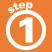Home    |    Teacher    |    Parents    |    Glossary    |    About UsIn the Language of Algebra, an equation is the basic number "sentence". An equation is a mathematical expression that contains an equals sign.

Click on the sentences below to translate them into equations.

 Homework Help | Algebra | The Language of AlgebraEmail this page to a friendSearch·  Definitions·  Order of Operations·  Writing equations·  Writing inequalitiesFirst Glance In Depth Examples WorkoutWriting equations